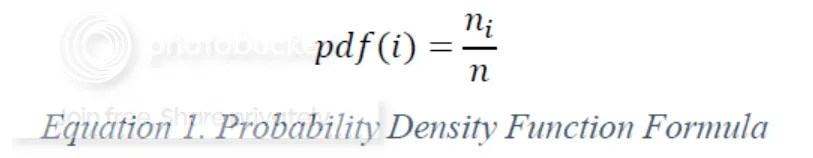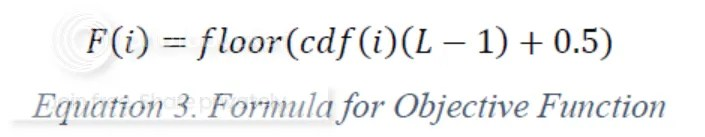# Histogram Equalization

Hi guys, after some time busy with my campus activities today I would like to update my blog. At the very recent article we have discussed about image histogram. One of the advantages is to create negative image. In this article I am going to discuss about the other advantages of image histogram. It has very popular in the image processing field. It is called Histogram Equalization.

What Histogram Equalization really is? Histogram equalization is a method that increases the contras of an image by increasing the dynamic range of intensity. This method works best for images that have less noise and do not contain regions of relative brightness or darkness. There are some term we have to know before understanding the algorithm. To ignite our motivation let’s take a look on that images below.Original CarHistogram Equalization Car

Yeah, you can see the purpose of Image Histogram equalization like I have mentioned above. It is time for the algorithm now. Consider we use a gray scale image as the input and there are L intensity levels for this type of image (in our case L =256). The first step is to go through all the pixels in image. For each intensity level, we need to count its occurrence and generate the probability density function.

Suppose there are n pixels in the image and if the intensity level I occurs ni times, so we can use the following equation to calculate pdf (probability density function).After obtaining the pdf, we use the pdf to generate the cumulative density function, use formula like in the following below.Our target is to derive a function F(i) so that for each intensity level i in the original imag, we can assign a new intensity level F(i) for it in the output image.Here, is my implementation in C++.

```void perform_histogram_equalization(struct Histo hist, int height, int width){
double s_hist_eq = { 0.0 }, sum_of_hist = { 0.0 };
long i, k, n, state_hst = { 0 };

n = width * height;

for (i = 0; i<256; i++){  // pdf of image
s_hist_eq[i] = (double)hist.data[i] / (double)n;
}
sum_of_hist = s_hist_eq;
for (i = 1; i<256; i++){        // cdf of image

sum_of_hist[i] = sum_of_hist[i - 1] + s_hist_eq[i];
}
outBuf = new unsigned char[height*width];
fill_n(transf.data, HISTOGRAM_SIZE, 0);
for (i = 0; i<height*width; i++){
k = inBuf[i];
outBuf[i] = (unsigned char)round(sum_of_hist[k] * 255.0);
transf.data[k] = outBuf[i];
}
}```

This algorithm also works in the color image. Just apply Histogram Equalization on each component independently. If you have any questions feel free to write down in comment. :)

## 2 thoughts on “Histogram Equalization”

1.Teddy Cahyadi

Great !!!, the result is very sharp and vividly clear. Hope you can apply this for phone camera

2.Jan Kristanto Post author

@Teddy Cahyadi : Thanks, in fact this has been implemented on many devices.